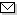Language:   Search:   Contact# Search and Browse the MSC 2000

Search:
Please note that in the second half of 2009, MSC2010 is deployed in the production of ZMATH and will become official standard in January 2010.

Enter a query and click »Search«...

42-XX Fourier analysis → ZMATH
42Axx Fourier analysis in one variable → ZMATH
42A05 Trigonometric polynomials, inequalities, extremal problems → ZMATH
42A10 Trigonometric approximation → ZMATH
42A15 Trigonometric interpolation → ZMATH
42A16 Fourier coefficients, Fourier series of functions with special properties, special Fourier series {For automorphic theory, see mainly 11F30} → ZMATH
42A20 Convergence and absolute convergence of Fourier and trigonometric series → ZMATH
42A24 Summability and absolute summability of Fourier and trigonometric series → ZMATH
42A32 Trigonometric series of special types (positive coefficients, monotonic coefficients, etc.) → ZMATH
42A38 Fourier and Fourier-Stieltjes transforms and other transforms of Fourier type → ZMATH
42A45 Multipliers → ZMATH
42A50 Conjugate functions, conjugate series, singular integrals → ZMATH
42A55 Lacunary series of trigonometric and other functions; Riesz products → ZMATH
42A61 Probabilistic methods → ZMATH
42A63 Uniqueness of trigonometric expansions, uniqueness of Fourier expansions, Riemann theory, localization → ZMATH
42A65 Completeness of sets of functions → ZMATH
42A70 Trigonometric moment problems → ZMATH
42A75 Classical almost periodic functions, mean periodic functions [See also 43A60] → ZMATH
42A82 Positive definite functions → ZMATH
42A85 Convolution, factorization → ZMATH
42A99 None of the above, but in this section → ZMATH

NewsAbel prize 2010I. M. Gelfand 1913-2009MSC2010

Copyright © 2020 Zentralblatt MATH | European Mathematical Society | FIZ Karlsruhe | Heidelberg Academy of Sciences